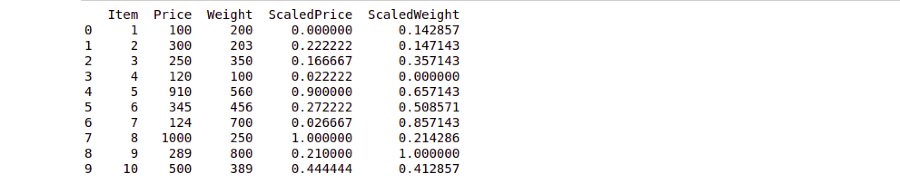# Python – Scaling numbers column by column with Pandas

• Last Updated : 25 Feb, 2021

Scaling numbers in machine learning is a common pre-processing technique to standardize the independent features present in the data in a fixed range. When applied to a Python sequence, such as a Pandas Series, scaling results in a new sequence such that your entire values in a column comes under a range. For example if the range is ( 0 ,1 ) your entire data within that column will be in the range 0,1 only.

Example:

```if the sequence is [1, 2, 3]
then the scaled sequence is [0, 0.5, 1]```

Application:

• In machine learning, scaling can improve the convergence speed of various algorithms.
• Often in machine learning, you will come across data sets with a huge variation, and it will be difficult for many machine learning models well on that data so in that case scaling helps to keep the data within a range.

Note: We will be using Scikit-learn in this article to scale the pandas dataframe.

Steps:

1. Import pandas and sklearn library in python.
2. Call the DataFrame constructor to return a new DataFrame.
3. Create an instance of sklearn.preprocessing.MinMaxScaler.
4. Call sklearn.preprocessing.MinMaxScaler.fit_transform(df[[column_name]]) to return the Pandas DataFrame df from the first step with the specified column min-max scaled.

Example 1 :

A very basic example of how MinMax

## Python3

 `# importing the required libraries ` `import` `pandas as pd ` `from` `sklearn.preprocessing ``import` `MinMaxScaler ` ` `  `# creating a dataframe for example ` `pd_data ``=` `pd.DataFrame({ ` `    ``"Item"``: [``1``, ``2``, ``3``, ``4``, ``5``, ``6``, ``7``, ``8``, ``9``, ``10``], ` `    ``"Price"``: [``100``, ``300``, ``250``, ``120``, ``910``, ``345``, ``124``, ``1000``, ``289``, ``500``] ` `}) ` ` `  `# Creating an instance of the sklearn.preprocessing.MinMaxScaler() ` `scaler ``=` `MinMaxScaler() ` ` `  `# Scaling the Price column of the created dataFrame and storing ` `# the result in ScaledPrice Column ` `pd_data[[``"ScaledPrice"``]] ``=` `scaler.fit_transform(pd_data[[``"Price"``]]) ` ` `  `print``(pd_data) `

Output :Example 2 :  You can also scale more than one pandas, DataFrame’s column at a time, you just have to pass the column names in the MinMaxScaler.fit_transform() function.

## Python3

 `# importing the required libraries ` `import` `pandas as pd ` `from` `sklearn.preprocessing ``import` `MinMaxScaler ` ` `  `# creating a dataframe for example ` `pd_data ``=` `pd.DataFrame({ ` `    ``"Item"``: [``1``, ``2``, ``3``, ``4``, ``5``, ``6``, ``7``, ``8``, ``9``, ``10``], ` `    ``"Price"``: [``100``, ``300``, ``250``, ``120``, ``910``, ``345``, ``124``, ``1000``, ``289``, ``500``], ` `    ``"Weight"``: [``200``, ``203``, ``350``, ``100``, ``560``, ``456``, ``700``, ``250``, ``800``, ``389``] ` `}) ` ` `  `# Creating an instance of the sklearn.preprocessing.MinMaxScaler() ` `scaler ``=` `MinMaxScaler() ` ` `  `# Scaling the Price column of the created dataFrame and storing ` `# the result in ScaledPrice Column ` `pd_data[[``"ScaledPrice"``, ``"ScaledWeight"``]] ``=` `scaler.fit_transform( ` `    ``pd_data[[``"Price"``, ``"Weight"``]]) ` ` `  `print``(pd_data) `

Output :Example 3: By default, the scale value used the class MinMaxScaler() is (0,1) but you can change it to any value you want as per your need.

## Python3

 `# importing the required libraries ` `import` `pandas as pd ` `from` `sklearn.preprocessing ``import` `MinMaxScaler ` ` `  `# creating a dataframe for example ` `pd_data ``=` `pd.DataFrame({ ` `    ``"Item"``: [``1``, ``2``, ``3``, ``4``, ``5``, ``6``, ``7``, ``8``, ``9``, ``10``], ` `    ``"Price"``: [``100``, ``300``, ``250``, ``120``, ``910``, ``345``, ``124``, ``1000``, ``289``, ``500``] ` `}) ` ` `  `# Creating an instance of the sklearn.preprocessing.MinMaxScaler() ` `# specifying the min and max value of the scale ` `scaler ``=` `MinMaxScaler(feature_range``=``(``20``, ``500``)) ` ` `  `# Scaling the Price column of the created dataFrame ` `# and storing the result in ScaledPrice Column ` `pd_data[[``"ScaledPrice"``]] ``=` `scaler.fit_transform(pd_data[[``"Price"``]]) ` ` `  `print``(pd_data) `

Output :My Personal Notes arrow_drop_up
Recommended Articles
Page :